## 6.3Vorticity transport

For an incompressible ﬂuid (with other simplifying assumptions), vorticity obeys the transport equation , Eq. (6.2 ). This is a typical advection-diﬀusion equation, similar to Eq. (2.65 ) for heat, which is expressed in terms of the local time derivative and advection in conservative form by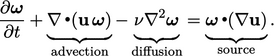(6.1)
Notably, Eq. (6.1 ) does not include a term in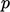. This is in contrast with conservation of (linear) momentum which can redistribute a perturbation in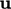instantaneously across all the domain through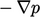, as discussed in Sec. 2.22 .

Instead, like heat, vorticity evolves locally only, with a range of inﬂuence limited by advective and diﬀusive transport, as discussed on page 126 .

Advection of vorticity is clearly illustrated by the smoke ring shown in Sec. 2.11 . Diﬀusion occurs by viscous torques transferring angular momentum between ﬂuid elements.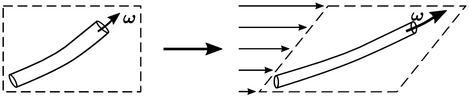The source of vorticity,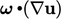is due to vortices changing shape under the inﬂuence of a velocity gradient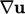. If a vortex is stretched, e.g. under shear as shown above, its radius decreases, so angular velocity, and thus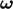, increases. Similarly,decreases if the vortex is compressed.

### The vorticity transport equation

For incompressible ﬂow with constant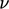(and zero, or constant, body force), vorticity obeys the following transport equation: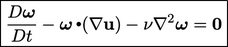(6.2)
The equation is derived from momentum conservation of a homogeneous, incompressible, Newtonian ﬂuid with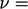constant and ignoring body forces, Eq. (2.49 ). Noting that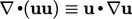due to Eq. (2.46 ) leads to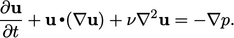(6.3)
The vorticity equation is derived from the curl (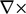) of Eq. (6.3 ) combined with the vorticity deﬁnition Eq. (2.37 ). The ﬁrst term is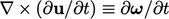.

Replacingand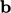by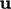in Eq. (2.72d ) and applying Eq. (2.37 ) gives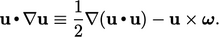(6.4)
The curl of the second term in Eq. (6.3 ) is expressed by the curl of Eq. (6.4 ) with Eq. (2.75a ), Eq. (2.73a ), Eq. (2.75e ) and Eq. (2.46 ), leading to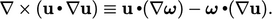(6.5)
The curl of the third term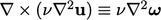by Eq. (2.74f ). The curl of the fourth term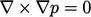by Eq. (2.75a ).

Combining all the terms and applying the material derivative Eq. (2.14 ),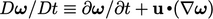to Eq. (6.5 ), leads to Eq. (6.2 ).

Notes on CFD: General Principles - 6.3 Vorticity transport